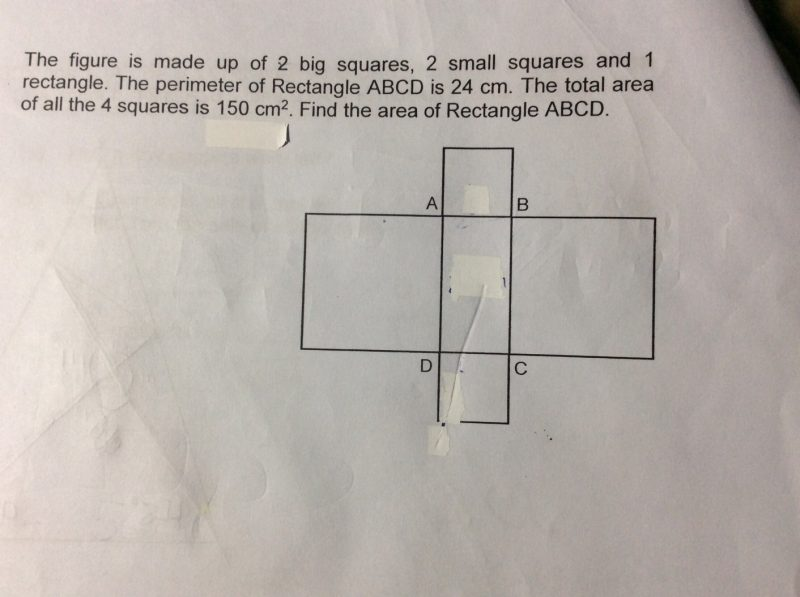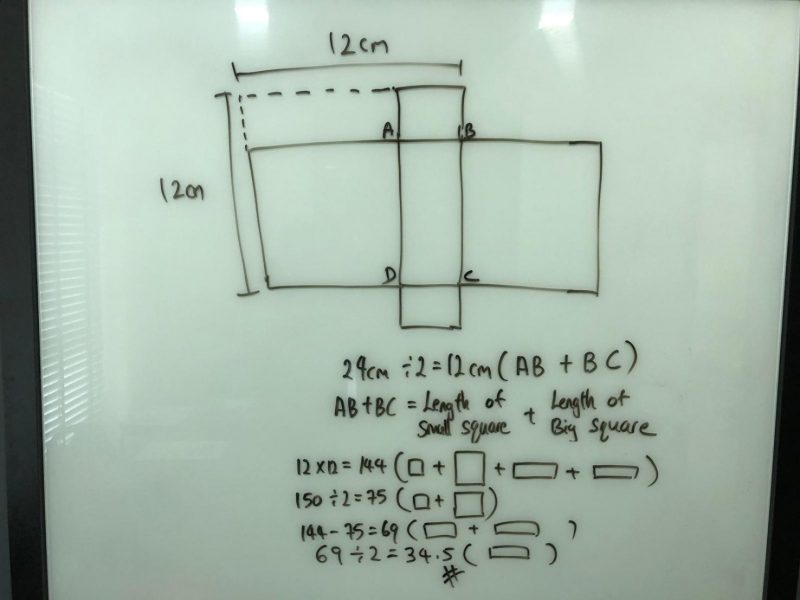# QuestionCan anyone please help me with this ?

Many thanks in advance

3 Answers

# Answer

I have tweaked the above question as follows : The figure is made up of 2 big squares, 2 small squares and 1 rectangle. The perimeter of the rectangle ABCD is 24 cm. The total area of all 4 squares is 148 sq cm. (a) What is the area of the rectangle? (b) What is the length of (i) one big square and (ii) one small square?

(a)
24/2 = 12 (total length of 1 big square and 1 small square)
148/2 = 74 (total are of 1 big square and 1 small square)
12 x 12 = 144
144 – 74 = 70
70/2 = 35

(b)
(ii)
74 = 49 + 25 = 7 x 7 + 5 x 5
length of one big square = 7 cm

(ii)
length of 1 small square = 5 cm

Ans : (a) 35 sq cm; (b)(i) 7 cm and (ii) 5 cm.

Note :
The total area of all 4 squares could also be changed to 160 sq cm.
(a)
24/2 = 12 (total length of 1 big square and 1 small square)
160/2 = 80 (total are of 1 big square and 1 small square)
12 x 12 = 144
144 – 80 = 64
64/2 = 32

(b)
(ii)
80 = 64 + 16 = 8 x 8 + 4 x 4
length of one big square = 8 cm

(ii)
length of 1 small square = 4 cm

Ans : (a) 32 sq cm; (b)(i) 8 cm and (ii) 4 cm.

0 Replies 0 Likes1 Reply 2 Likes

I like your solution.

0 Replies 0 Likes

Perimeter of ABCD = 2AB + 2AD = 24 cm

=> 2 (AB + AD ) = 24

=>   AB + AD = 12
Area of 4 squares = 2AB2 + 2AD2 = 150

=> 2 (AB2 + AD2 ) = 150

=>   AB2 + AD2 = 75

(AB + AD)2 = 12=144

(AB + AD)2 = AB2 + AD2 +2 AB x AD

(AB + AD)2 = 75 +2 AB x AD

Therefore,

75 +2 AB x AD = 144

2 AB x AD = 144 – 75

2 AB x AD = 69

AB x AD = 34.5 cm

Area of ABCD = AB x AD = 34.5 cm

0 Replies 0 Likes